# Scalar

In physics, the word scalar is often used to refer simply to a real or complex number. In other contexts, where vectors and tensors may also play a role, a scalar is defined by its transformation properties.

In order to explain the second definition of a scalar, it must be noted that most physical objects belong to—or are associated with—some linear space with inner product. The algebraic form of a physical quantity is—explicitly or implicitly—defined with respect to an orthonormal basis of this space. When a new orthonormal basis of the space is chosen, the algebraic form of a quantity expressed with respect to this new basis is in general different from the algebraic form of the same quantity with respect to the old basis. One expresses this by stating that physical quantities transform under change of reference frame (i.e., under change of basis of the space).

The transformation from one orthonormal basis of a linear space to another is called unitary or orthogonal, depending on whether the space is complex or real (if the space is real and 3-dimensional it is called a rotation). The determinant of a unitary transformation is a complex number of absolute value unity, that is, the determinant of a unitary transformation is on the unit circle in the complex plane. Transformations with determinant equal to 1 are called unimodular. Orthogonal (real) matrices clearly have determinant ±1.

The second kind of scalars then are quantities that are invariant under unimodular orthogonal basis transformations. That is, unlike physical quantities as vectors and tensors, scalars do not change under such a basis transformation. Two kinds of scalars are distinguished: proper scalars, which are invariant under all orthogonal transformations and pseudo-scalars, which are invariant under unimodular orthogonal transformations and obtain a minus sign upon transformation by non-unimodular orthogonal transformations (those with determinant equal to −1). With respect to a given orthonormal reference frame and expressed in a suitable set of units, such as SI units, a scalar is represented by a single number. The essential property of a scalar is that this number is the same with respect to all frames that are related to each other by unimodular orthogonal transformations.

## Example

Examples of scalars of the first kind (no reference to a frame) are the number 3.14, 2exp[-i 300], the mass of the Earth in kilogram, and the elementary charge in Coulomb.

For an example of scalars of the second kind, we consider two elements,$\vec{r},\; \vec{s}$ in ℝ3. These vectors may have some physical meaning, for example, direction and strength of an electric and magnetic field, or velocities of two particles. Let the following be a basis of ℝ3,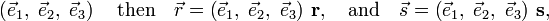$\left( \vec{e}_1,\; \vec{e}_2, \;\vec{e}_3 \right)\quad\hbox{then}\quad \vec{r} = \left( \vec{e}_1,\; \vec{e}_2, \;\vec{e}_3 \right)\, \mathbf{r},\quad\hbox{and}\quad \vec{s} = \left( \vec{e}_1,\; \vec{e}_2, \;\vec{e}_3 \right)\,\mathbf{s} ,$

where the column vectors (bold) are$\mathbf{r} \equiv \begin{pmatrix} r_1 \\ r_2\\r_3 \end{pmatrix}\quad\hbox{and}\quad \mathbf{s} \equiv \begin{pmatrix} s_1 \\ s_2\\s_3 \end{pmatrix},\;$

and the matrix-matrix product is implied,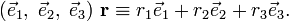$\left( \vec{e}_1,\; \vec{e}_2, \;\vec{e}_3 \right)\, \mathbf{r} \equiv r_1 \vec{e}_1 + r_2 \vec{e}_2 +r_3\vec{e}_3.$

The column vectors r and s represent algebraically the physical quantities$\vec{r},\; \vec{s}$ with respect to the given basis.

Choose now another basis of the same space$\big( \vec{f}_1,\; \vec{f}_2, \;\vec{f}_3 \big)\quad\hbox{with}\quad \big( \vec{e}_1,\; \vec{e}_2, \;\vec{e}_3 \big) = \big( \vec{f}_1,\; \vec{f}_2, \;\vec{f}_3 \big)\mathbf{B}$

The 3×3 matrix B is assumed to be an orthogonal matrix (transforms orthonormal bases into each other). Clearly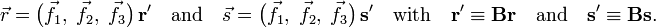$\vec{r} = \big( \vec{f}_1,\; \vec{f}_2, \;\vec{f}_3 \big)\, \mathbf{r'}\quad\hbox{and}\quad \vec{s} = \big( \vec{f}_1,\; \vec{f}_2, \;\vec{f}_3 \big)\, \mathbf{s'} \quad\hbox{with}\quad \mathbf{r'} \equiv \mathbf{Br} \quad\hbox{and}\quad\mathbf{s'} \equiv \mathbf{Bs}.$

The columns r', s' represent the same vectors$\vec{r},\; \vec{s}$ with respect to the new basis. They have another form, or more accurately, their three components have new values.

To construct a proper scalar (transformation invariant), we consider the inner product. The inner product is not a member of ℝ3, but is clearly associated with it. (Formally it is a bilinear map of the Cartesian product ℝ3×ℝ3 into ℝ). The inner product satisfies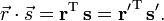$\vec{r}\cdot\vec{s} = \mathbf{r}^\mathrm{T}\, \mathbf{s} = \mathbf{r'}^\mathrm{T}\, \mathbf{s'}.$

The second equality follows in short-hand notation thus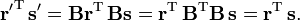$\mathbf{r'}^\mathrm{T}\, \mathbf{s'} = \mathbf{Br}^\mathrm{T}\, \mathbf{Bs} = \mathbf{r}^\mathrm{T}\, \mathbf{B}^\mathrm{T}\mathbf{B}\, \mathbf{s} = \mathbf{r}^\mathrm{T}\, \mathbf{s}.$

In long-hand notation,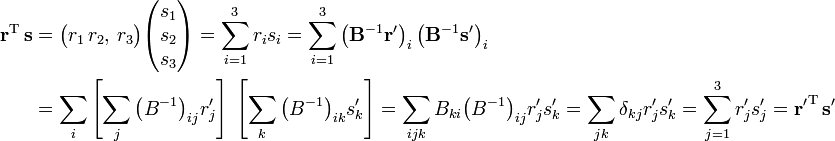\begin{align} \mathbf{r}^\mathrm{T}\, \mathbf{s} &= \big(r_1\, r_2, \,r_3\big)\begin{pmatrix} s_1\\s_2\\s_3\\ \end{pmatrix} = \sum_{i=1}^3 r_i s_i = \sum_{i=1}^3 \left(\mathbf{B}^{-1} \mathbf{r'}\right)_i \left(\mathbf{B}^{-1} \mathbf{s'}\right)_i \\ &=\sum_{i}\left[\sum_j \big(B^{-1}\big)_{ij} r'_j\right] \; \left[\sum_k \big(B^{-1}\big)_{ik} s'_k \right] =\sum_{ijk} B_{ki} \big(B^{-1}\big)_{ij} r'_j s'_k = \sum_{jk} \delta_{kj} r'_j s'_k = \sum_{j=1}^3 r'_j s'_j =\mathbf{r'}^\mathrm{T}\, \mathbf{s'} \end{align}

In both notations it is used that B is orthonormal,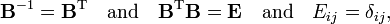$\mathbf{B}^{-1} = \mathbf{B}^\mathrm{T}\quad \hbox{and} \quad \mathbf{B}^\mathrm{T}\mathbf{B} = \mathbf{E}\quad\hbox{and}\quad E_{ij} = \delta_{ij},$

where δij is the Kronecker delta. The inner product of$\vec{r},\; \vec{s}$ does not depend on the basis used to express the vectors, it is a proper scalar. This proof holds for proper (unimodular) rotations and for improper (determinant = −1) rotations.

As an example of a pseudo-scalar the following triple product may be given$\vec{r} \cdot \left( \vec{s}\times\vec{t}\,\right).$

The product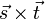$\vec{s}\times\vec{t}$ stands for a cross product, it is again an element of ℝ3 (sometimes referred to a pseudo vector) and hence the triple product, being an inner product, is a scalar. Remember that the improper rotations can be written as a product of a proper rotation times inversion. Under inversion the three vectors go into minus themselves and the triple product obtains the factor (−1)3 = −1 and in total the triple product is a pseudo-scalar.

## Scalar functions

Usually the term "scalar function" is used for a single-valued function on a certain space that map elements of the space pointwise into ℝ (real functions) or ℂ (complex functions). Scalar functions must be distinguished from vector functions that have more values—their components. In general vector functions map a space into ℝn or ℂn, n > 1.

If Φ is a scalar function on ℝ3, we can define the operator ℛ by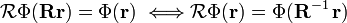$\mathcal{R} \Phi (\mathbf{R} \mathbf{r}) = \Phi(\mathbf{r}) \;\Longleftrightarrow \mathcal{R} \Phi (\mathbf{r}) = \Phi(\mathbf{R}^{-1}\,\mathbf{r})$

where R is the matrix of a non-singular map ℝ3 → ℝ3. The "if and only if arrow" follows because the relations are true for all r, including R−1r and Rr

A scalar function on ℝ3 is spherically symmetric if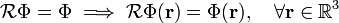$\mathcal{R} \Phi = \Phi \; \Longrightarrow \; \mathcal{R} \Phi(\mathbf{r}) = \Phi(\mathbf{r}), \quad \forall \mathbf{r} \in \mathbb{R}^3$

where an orthogonal (length preserving) matrix R appears in the definition of ℛ.